10.2 C
New York
Sunday, December 3, 2023
HomeProgrammingThe 20 Best Matlab Books For Beginner and Expert Developers

# The 20 Best Matlab Books For Beginner and Expert Developers

MATLAB is short for Matrix Laboratory. MATLAB is the simplest and most profitable computing climate for researchers, scientists, and engineers. It incorporates the MATLAB language, the main programming language devoted to numerical and specialized computing. You can utilize MATLAB for a scope of utilizations, including Artificial Intelligence, signal preparing and interchanges, picture and video handling, deep learning, control frameworks, test and estimation, and computational science. Thus, in this modern era, it has become very crucial to learn MatLab, and for the appropriate guidance, an adequate set of MatLab books is inevitably important.

## Best Matlab Books For Beginner and Expert

We all know how challenging it can be for someone to learn a new programming language. But Matlab is not only easy to learn but also fun to work with. This is just the language that mathematical minds have been looking for for years now.

Since the invention of this programming language, it has been a total game-changer for students, scientists, and researchers. Therefore, to make your learning more fun and less strenuous, we have given you a list of the top commonly used MatLab books.

### 1. Process Control: A First Course with MATLAB

If you ask me about a language that would cover mathematical and technical computing, then the name would be Matlab. The amazing thing about this language is it has a wide range of application fields in industrial engineering.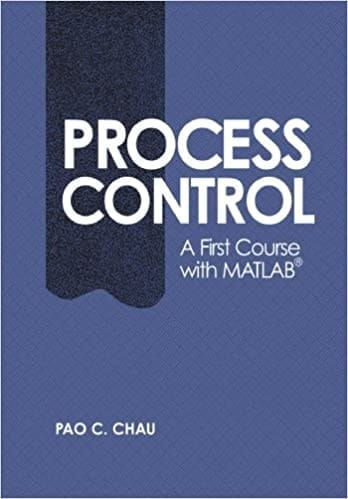Process Control – A First Course with MATLAB’ is one of those Matlab books. In this book, you will find Matlab’s fundamentals that would be needed in the chemical engineering sector. You will find a total of 10 chapters along with a Matlab tutorial session and practice problem.

This book has made sure of your complete understanding of this topic. Introduction to Matlab, preliminaries of mathematics, PID control system, response analysis, etc., are some of the topics from this book.

### 2. Digital signal and image processing using MATLAB

If you are someone from the software sector, you must have heard about Matlab and its versatile movement. Matlab is a high-level fourth-generation language that has become so popular for its visualization and numerical computation.There is multidimensional use of Matlab, and I will introduce with you one of these amazing Matlab books named ‘Digital Signal and Image Processing Using Matlab.’ This book covers all the necessary topics that you might need to learn for signal and image processing.

You will find an introductory discussion about Matlab, a detailed discussion about the deterministic signal, a detailed discussion about random signals, digital communication, and lots more inside this book of 14 chapters.

### 3. MATLAB for Dummies

Among beginners, Matlab has gradually become a famous environment and language in recent times. Another reason for this language being so popular is that it has its necessity almost in every field. Not only for data manipulation but also for graphing and plotting data, Matlab is an amazing tool.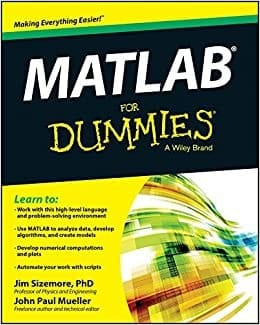‘Matlab for dummies’ is a perfect guideline for this computational language. Here you will find details from installation to plotting, visualizing, and practical implementation. Inside the book, you will find 6 main parts in total.

These 6 parts have been divided into 18 chapters. Matlab, data plotting and manipulation, advanced techniques of Matlab, some specified Matlab applications, etc., are some contents from this book.

### 4. Advanced Image and Video Processing Using MATLAB

Matlab is a very famous language for people of almost every sector in recent days. Among numerous amazing features, image and video processing is something very interesting and useful feature you can do with Matlab. This book has tried to cover both beginner and advanced level contents of image processing.

The authors have tried to overcome all those traditional issues that scientists and professionals have been facing for years and tried to enrich this book with many new theories and methods of learning and understanding even better.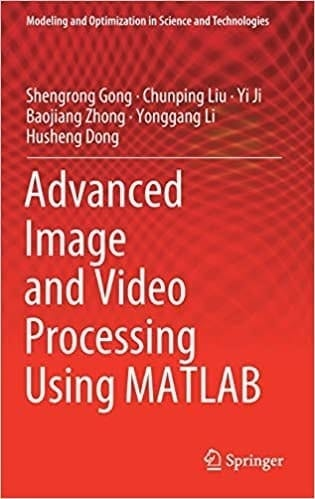This is a book of mainly three parts and 14 subparts or chapters. Brief introduction of the topics, image correction and segmentation, image fusion, and watermarking, advanced video processing techniques are some contents from the book.

### 5. Practical Matlab Basics for Engineers

If we mention some of the most famous programming languages of this recent time, then Matlab would be one of them. Especially if you are an engineer, then learning Matlab is a necessity in your field. Matlab’s beauty is that this language is not at all difficult to learn, and it has many application fields.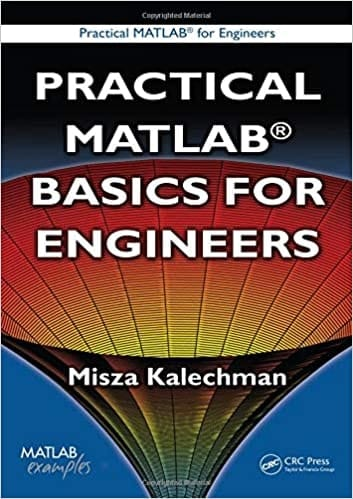To know about Matlab even better, let me introduce you to one of the incredible Matlab books named ‘Practical Matlab for engineers’. No matter which stage of learning you are in, this book will help you with your journey.

Topics like Matlab with the trend and industry, basic mathematical terms using Matlab, printing, and plotting, complex number studies have been covered in this book. In a nutshell, having this book can complete your journey with Matlab smoothly and almost effortlessly.

### 6. Introduction to scientific computing – twelve projects with MATLAB

Matlab is a language that has a huge range of usages in the field of software and engineering. No matter if it’s about industrial engineering, numerical computation, image processing, or scientific computing, Matlab just fits in perfectly everywhere.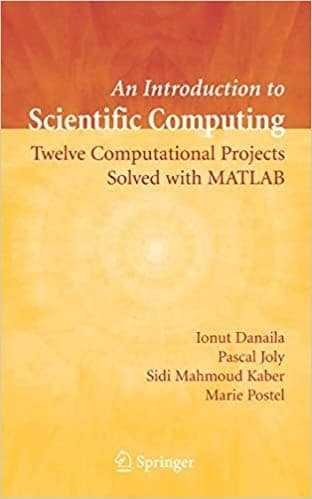Here I am going to talk about a book of introductory level scientific computing Matlab book. This book is both a discussion and a practical problem-solving-based book that will help you complete your learning. You will find a total of 12 chapters in this book. Some of the topics discussed are the nonlinear differential equation, Approximation of polynomial, signal processing analysis, geometrical design, etc.

### 7. Functional data analysis with R and MATLAB

Matlab is very easy to learn and one of the most important languages in the engineering field. This language especially focuses on mathematical terms such as the matrix, array, function, etc. To analyze functional data, if you want a comfortable and flexible language, then Matlab ought to be the first choice.This book named ‘Functional data analysis with R and Matlab’ is here to make your functional data analysis even smoother. Another great deal about this book is that it covers both Matlab and R language together to have a better idea about both of them. In this book of 11 chapters, you will find topics like a brief introduction to functional data analysis, comparison between Matlab and R, Smoothing, registration, and so on.

### 8. Optimization in Practice with MATLAB

If you are new in the field of programming and engineering and would ask for one language that can provide you multi-dimensional supports for every field you wish to explore further, then you will be suggested Matlab.

To start with anything, we search for a book first. ‘Optimization with practice using Matlab’ is one of those unique books that will provide you with an amazing approach toward learning optimization. No matter whether you are a pro or a noob, this book would be suitable for all.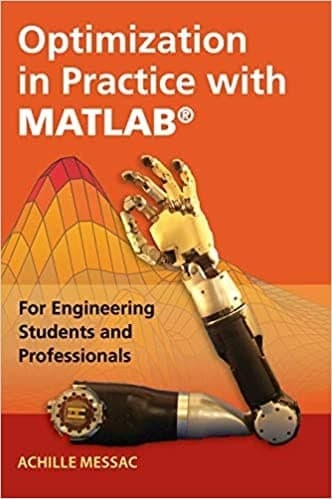This book of 19 chapters will provide you with all kinds of practical knowledge you might need in the real world. Preliminaries of mathematics, a fascinating world of optimization, multiobjective optimization, linear programming, and evolutionary algorithms are some of the contents of this amazing book.

### 9. A Guide to MATLAB Object-Oriented Programming

Object-oriented programming has a huge demand in the language field. Nothing can be more fascinating and fun to learn if we can create a combination of object-oriented and Matlab language. Matlab is such a language that has multi-dimensional use, and one can easily learn this language.‘A guide to Matlab object-oriented programming’ is such a book that will provide you with a proper guideline of programming with Matlab. This book contains some really important content that would clear your understanding more.

Here you will find 3 main parts and 24 chapters in total. Some of the covered topics are an introduction to the whole idea, Matlab requirements, initial value specification, strategies of advanced stage, and lots more.

### 10. Essential MATLAB for Engineers and Scientists

Matlab is not only a language of engineers but also for scientists. From this statement, we can guess how interesting this language can be. The best part about this language is that this is not at all hard to learn. For students of engineering, mathematics, and scientists, Matlab is a very useful language.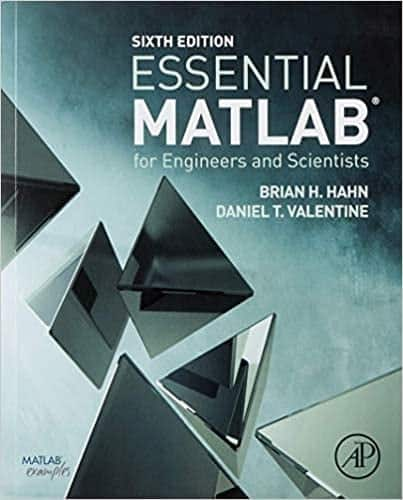To guide you through your journey with Matlab, an introductory book would be incredible. To help you with that, I am introducing to you a book named ‘Essential Matlab for engineers and scientists. This is an introductory book for Matlab learners, but it has this complete suggestion.

This book has 2 main parts and 17 chapters. The first part talks about the essentials like introduction and fundamental basics, and the second part is an application such as a dynamical system, numeric methods, etc.

### 11. Advanced Mathematics and Mechanics Applications Using MATLAB

To work with mathematical terms for students of any field, Matlab is the name that comes to mind first. Matlab covers such a huge field of topics, but it is most suitable for mathematical terms. This language is full of amazing features and can easily handle real-life problems.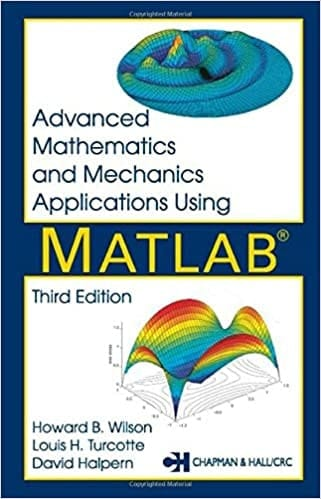For advanced learners ‘Advanced mathematics and mechanics application using Matlab’ is a great book. This book analyzes the various applications of Matlab, covering the topics of mathematics and mechanics.

This book not only covers the theory parts but also has problem-solving and analyzing parts. This book of 13 chapters covers topics like brief introduction, concepts of linear algebra, interpolation and differentiation, analytic function application, etc.

### 12. Numerical Methods in Engineering With MATLAB

The efficiency of Matlab in the field of mathematical and scientific analysis is quite large. This is the language that covers all possible mathematical terms along with graphing. Overall we can say this language is a versatile one that has its place almost everywhere.‘Numerical method with engineering using Matlab’ is one of those books that cover all those relevant numerical analyses that an engineering student might need. The representations of topics in this book are so clear and precise that a learner would find it easy and interesting to learn Matlab. There are 10 chapters covering topics like introduction, linear algebra equation system, numerical integration, and differentiation, etc.

### 13. Accelerating MATLAB with GPU Computing

Matlab can amazingly do mathematical and data annalistic works. Beyond all these basics, Matlab is also being used in product deployment in the computational field. Other than these, Matlab also provides high-level functions for GPU simulation.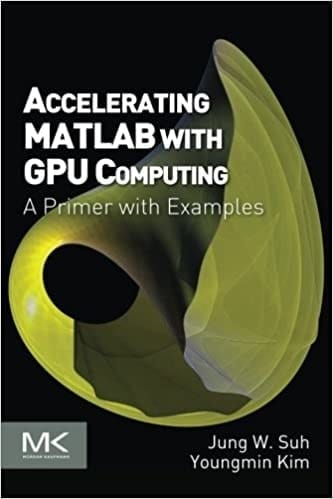I have this awesome book for you named ‘Accelerating Matlab with GPU computing’ to explore more on this topic. This book would be efficient for everyone interested in Matlab and wants to explore this field more intensely.

As a reader, you will find 8 chapters total in this book. I want to mention some of the topics from here are Matlab without the GPU, required configuration for Matlab, coding details, code libraries, and examples.

### 14. Solving ODEs with MATLAB

Matlab is a language that has been widely used for solving different mathematics-based problems such as matrix, linear algebra, functions, differential equations, and whatnot. In simple words, to solve mathematical problems, Matlab is the best prop.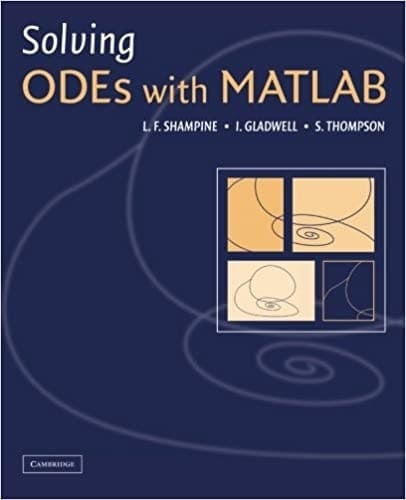If you are working with an ordinary differential equation, then I have a perfect Matlab book for you. ‘Solving ODEs with Matlab’ is that perfect book. This book not only discusses the topics but also provides a problem-solving session after finishing every chapter.

We can find to have four different chapters of content in this book. Those are- Starting, initial value problem discussion, Boundary value problem, and delay differential equation. Under these 4 chapters, you will find some small parts of that topic which will make your learning easier.

### 15. MATLAB for beginners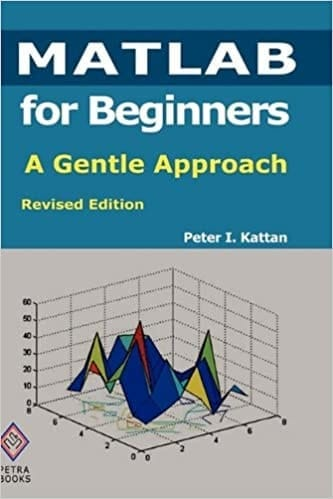Matlab is one language that can be used anywhere and everywhere. So learning about this tool would be beneficial for anyone. If you start with this language, then as a beginner, the first thing you might need is proper guidance.

So to provide you that I am introducing you ‘Matlab for beginner’. This book would undoubtedly be a great companion and guidance for you. Moreover, the contents are being designed so flawlessly that you need not suffer much with this topic, and you will be able to learn it quite easily.

### 16. MATLAB Graphics and Data Visualization Cookbook

It is impossible and unnecessary to explain in words how useful and efficient language Matlab is. We can find it useful almost in every professional sector and which is at the same time quite convenient and fascinating. Another great thing about Matlab is that you can visualize and graph data precisely with Matlab.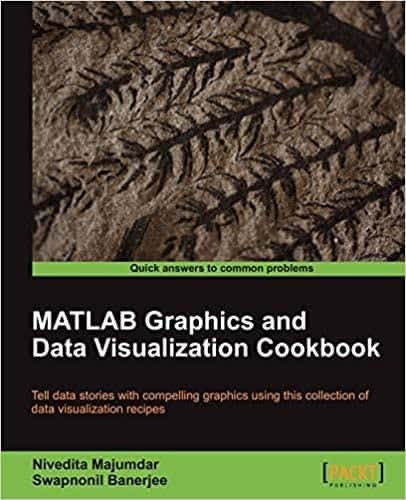This book is an absolute gem in the crowd of Matlab books. Here you will be guided with some stepwise application instructions to make you more comfortable with it. The remedies and recipes that this book provides are quite easier for your better understanding.

You will find 8 chapters full of content in this book. Customizing Matlab graphics elements, two-dimensional data displays, creating interactive graphics and animation, and so many interesting topics can be found in the book.

### 17. Business, Economics, and Finance with Matlab

We are all more or less familiar with the Matlab language and its application field, how variety this language offers. Another interesting sector of Matlab is its use in the business and economy. Yes, not only is science and engineering students but also for the business community, Matlab is a popular name.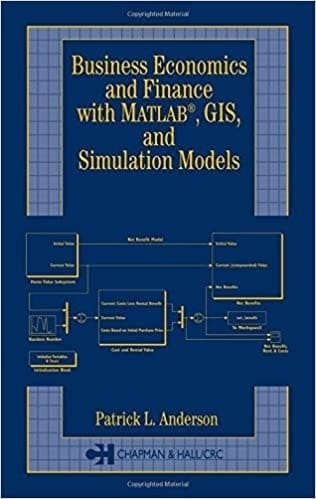I have this great book named ‘ Business economics and finance with Matlab, GIS, and simulation models’ to start with Matlab. From this book’s name, you can guess what this book exactly discusses and what it provides.

You will find a total of 17 chapters here. Some of the book’s topics are named the use of this book, the relation of Matlab with business and economics, economic and fiscal impact model, fuzzy logic and its business application, and many more.

### 18. An Introduction to Programming and Numerical Methods in MATLAB

Matlab is a language that has been designed for working with mathematics. This high level of language has been popular these days for its usefulness in almost all fields. Moreover, this language is not that complex to work with.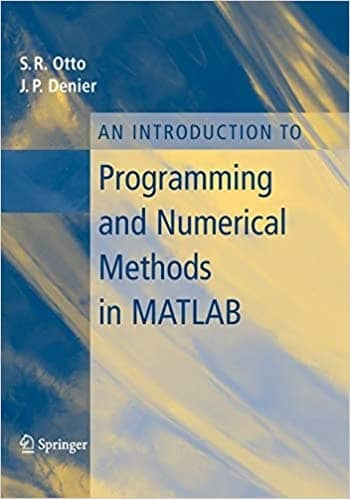In this book, the author has aimed that this book will work as a Matlab command reference source for a learner. For students, a book like this is helpful. This will provide a basic introduction to numerical methods along with some exercise problems for you.

Here you will find 9 different chapters. Simple Matlab calculation, Script and function writing, discussion on a conditional statement, numerical integration, and simulations are some of this book’s contents.

### 19. Numerical analysis using MATLAB and Excel

To work with numerical data and analysis them required a proper programming language. Matlab is the name of that language. Not only for numerical one but also in every sector from business to scientific research Matlab can be found everywhere.To start with Matlab, a perfect book would be really helpful. For that purpose, I am presenting this wonderful book named ‘Numerical analysis using Matlab and excel’. As you can see, this is the third edition of the book, so this one is more improvised and advanced.

As a reader, you will get a theoretical analysis of each topic and a comprehensive summary of every chapter. Here you will find 16 chapters and some of them are an introduction to Matlab, discussion of matrices and determinants, finite difference, interpolation, partial function expansion, etc.

### 20. Learning MATLAB

Engineers, mathematicians, and scientists don’t have to explain how useful and important is learning Matlab. This multipurpose language has been used in every sector. If you can become a pro at this, I can guarantee there are so many fields for you to explore.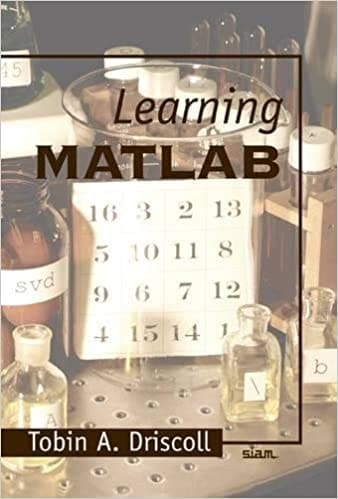So for a beginner Matlab learner, a perfect book cannot go wrong. This book ‘Learning Matlab’ is such an amazing book for beginners. You will find 7 chapters of content here. Introduction to Matlab, script, function, array, matrices, graphics, advanced learning techniques, scientific computing are some of the contents. Overall I can say this book would be a great companion for your journey with Matlab.

## Some Final Words

MATLAB is an exclusive multi-worldview programming language and mathematical figuring climate created by MathWorks. MATLAB permits network controls or matrix manipulations, plotting capacities and information, execution of calculations and algorithms, the formation of UIs, and interfacing with programs written in different dialects.

We really hope that this list of MatLab books that we have suggested above will be useful to you. Just like any other skill, learning a new language like MatLab will require your time and a bit of effort.

If you are willing to give that, then you have already come halfway through your learning journey. Finally, if you have been succored by the information we have provided, please do not hesitate to share it with your friends and family. Best of luck!Mehedi Hasan
Mehedi Hasan is a passionate enthusiast for technology. He admires all things tech and loves to help others understand the fundamentals of Linux, servers, networking, and computer security in an understandable way without overwhelming beginners. His articles are carefully crafted with this goal in mind - making complex topics more accessible.